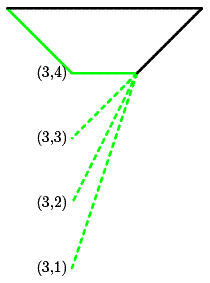### Problem Statement

An array of integers is called convex if all of the following apply:

• It contains an even number of elements.
• It contains between 6 and 50 elements, inclusive.
• Each element is an integer between 1 and 50, inclusive. Interpret the integers as the coordinates of n points: x_1, y_1, x_2, y_2, ..., x_n, y_n (in this order).
• These n points are vertices of a convex polygon.
• The vertices are listed in either clockwise or counterclockwise order.
• No three consecutive vertices lie on the same straight line (when saying three consecutive vertices we also mean vertices number N, 1 and 2 and vertices number N-1, N and 1).

Given a int[] beginning, find the shortest int[] that will produce a valid convex array when appended to the end of beginning. If there are multiple such int[]s, return the one among them that comes first lexicographically. If there is no such int[], return a int[] containing a single element -1.

### Definition

 Class: ConvexArray Method: getEnding Parameters: int[] Returns: int[] Method signature: int[] getEnding(int[] beginning) (be sure your method is public)

### Constraints

-beginning will contain between 0 and 50 elements, inclusive.
-Each element of beginning will be between 1 and 50, inclusive.

### Examples

0)

 `{1, 1, 2, 2}`
`Returns: {1, 2 }`
 The polygon must contain at least 3 points, so we need to add at least one more. Adding (1, 1) would give us a segment, not a polygon, thus we stick to the next lexicographical point which is (1, 2).
1)

 `{5, 6, 6}`
`Returns: {1, 1, 1 }`
 It is possible that the x coordinate is given and you have to choose the y coordinate.
2)

 `{1, 3}`
`Returns: {1, 1, 2, 1 }`
 (1, 1, 1, X) would form a segment, not a triangle. Move on to the next lexicographical quadruple, (1, 1, 2, 1).
3)

 `{2, 5, 5, 5, 4, 4, 3}`
`Returns: {4 }`We need to find the y coordinate for the last vertex that will generate a convex polygon. Points (3, 1) and (3, 2) would make the polygon non-convex. Point (3, 3) would break the rules because there would be three consecutive vertices on the same straight line. Point (3, 4) is the only valid choice.
4)

 `{1, 1, 2, 3, 3, 1, 1, 2, 3}`
`Returns: {-1 }`No matter what the last y-coordinate is, the edge (1, 1)-(2, 3) intersects the edge (3, 1)-(1, 2), so there is no convex polygon possible.

#### Problem url:

http://www.topcoder.com/stat?c=problem_statement&pm=7877

#### Problem stats url:

http://www.topcoder.com/tc?module=ProblemDetail&rd=10772&pm=7877

darnley

#### Testers:

PabloGilberto , Olexiy , lovro , ivan_metelsky

Geometry, Search